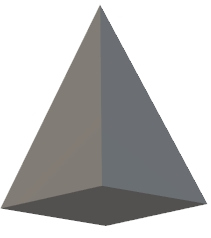# Program for volume of Pyramid in C++

Given with sides depending upon the type of base of pyramid the task is to calculate the volume of pyramid.

Pyramid is a 3-D figure whose outer surfaces are triangular meeting at the common point forming the sharp edge of pyramid. Volume of pyramid depends upon the type of base it will have.

There are different types of base a pyramid can be made up of, like −

Triangular −It means pyramid will have triangular base, than the volume of pyramid will be

Formula - : (1/6) * a * b * h

Square −It means pyramid will have square base, than the volume of pyramid will be

Formula - : (1/3) * (b^2) * h

Pentagonal −It means pyramid will have pentagonal base, than the volume of pyramid will be

formula - : (5/6) * a * b * h

Hexagonal −It means pyramid will have hexagonal base, than the volume of pyramid will be

formula - : a * b * h

## Example

Input-: a=4 b=2 h=10
Output-: Volume of pyramid with triangular base is 13.328
Volume of pyramid with square base is 13.2
Volume of pyramid with pentagonal base is 66.4
Volume of pyramid with hexagonal base is 80

Given below is the pyramid with square base## Algorithm

Start
Step 1 -> Declare function to find the volume of triangular pyramid
float volumeTriangular(int a, int b, int h)
Declare variable float volume = (0.1666) * a * b * h
return volume
step 2 -> Declare Function to find the volume of square pyramid
float volumeSquare(int b, int h)
declare and set float volume = (0.33) * b * b * h
return volume
Step 3 -> Declare Function to find the volume of pentagonal pyramid
float volumePentagonal(int a, int b, int h)
declare and set float volume = (0.83) * a * b * h
return volume
Step 4 -> Declare Function to find the volume of hexagonal pyramid
float volumeHexagonal(int a, int b, int h)
declare and set float volume = a * b * h
return volume
Step 5 -> In main()
Declare variables as int b = 2, h = 10, a = 4
Call volumeTriangular(a, b, h)
Call volumeSquare(b,h)
Call volumePentagonal(a, b, h)
Call volumeHexagonal(a, b, h)
Stop

## Example

#include <bits/stdc++.h>
using namespace std;

// Function to find the volume of triangular pyramid
float volumeTriangular(int a, int b, int h){
float volume = (0.1666) * a * b * h;
return volume;
}
// Function to find the volume of square pyramid
float volumeSquare(int b, int h){
float volume = (0.33) * b * b * h;
return volume;
}
// Function to find the volume of pentagonal pyramid
float volumePentagonal(int a, int b, int h){
float volume = (0.83) * a * b * h;
return volume;
}
// Function to find the volume of hexagonal pyramid
float volumeHexagonal(int a, int b, int h){
float volume = a * b * h;
return volume;
}
int main(){
int b = 2, h = 10, a = 4;
cout << "Volume of pyramid with triangular base is "<<volumeTriangular(a, b, h)<<endl;
cout << "Volume of pyramid with square base is "<<volumeSquare(b, h)<< endl;
cout << "Volume of pyramid with pentagonal base is "<<volumePentagonal(a, b, h)<< endl;
cout << "Volume of pyramid with hexagonal base is "<<volumeHexagonal(a, b, h);
return 0;
}

## Output

Volume of pyramid with triangular base is 13.328
Volume of pyramid with square base is 13.2
Volume of pyramid with pentagonal base is 66.4
Volume of pyramid with hexagonal base is 80